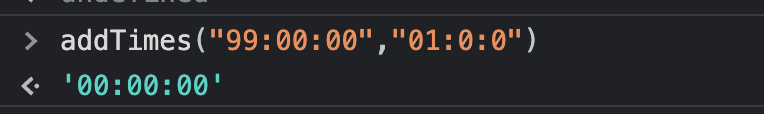Instantly share code, notes, and snippets.

Last active Jan 17, 2023
Add two string time values (HH:mm:ss) with javascript
This file contains bidirectional Unicode text that may be interpreted or compiled differently than what appears below. To review, open the file in an editor that reveals hidden Unicode characters. Learn more about bidirectional Unicode characters
 /** * Add two string time values (HH:mm:ss) with javascript * * Usage: * > addTimes('04:20:10', '21:15:10'); * > "25:35:20" * > addTimes('04:35:10', '21:35:10'); * > "26:10:20" * > addTimes('30:59', '17:10'); * > "48:09:00" * > addTimes('19:30:00', '00:30:00'); * > "20:00:00" * * @param {String} startTime String time format * @param {String} endTime String time format * @returns {String} */ function addTimes (startTime, endTime) { var times = [ 0, 0, 0 ] var max = times.length var a = (startTime || '').split(':') var b = (endTime || '').split(':') // normalize time values for (var i = 0; i < max; i++) { a[i] = isNaN(parseInt(a[i])) ? 0 : parseInt(a[i]) b[i] = isNaN(parseInt(b[i])) ? 0 : parseInt(b[i]) } // store time values for (var i = 0; i < max; i++) { times[i] = a[i] + b[i] } var hours = times var minutes = times var seconds = times if (seconds >= 60) { var m = (seconds / 60) << 0 minutes += m seconds -= 60 * m } if (minutes >= 60) { var h = (minutes / 60) << 0 hours += h minutes -= 60 * h } return ('0' + hours).slice(-2) + ':' + ('0' + minutes).slice(-2) + ':' + ('0' + seconds).slice(-2) }

### grauziitisos commented Apr 6, 2022

Actually some company out there thinks the opposite
( regarding what is the meaning of shorter form ).

from opensource projects I can name ffmpeg, for example.

that shorter form means there are only the smaller units not the bigger ones :-D

So to turn that mode on here:
//4 lines:
//beggining:

``````function addTimes (startTime, endTime, shortMeansSmaller) {
var s = shortMeansSmaller;
``````

// and then line 8 as is (i cite here it as first line)

``````//8th line:
var b = (endTime || '').split(':')
if(s) { if(a.length <3){var l = a.length; var t=[]; for(var i=0; i<l; i++) t.push(a.pop()); for(var i=0; i<3-l; i++) a.push(0); for(var i=0; i<l; i++) a.push(t.pop());}
if(b.length <3){var l = b.length; var t=[]; for(var i=0; i<l; i++) t.push(b.pop()); for(var i=0; i<3-l; i++) b.push(0); for(var i=0; i<l; i++) b.push(t.pop()); }
}

``````

Then with the data:

``````var tm = ['13:22', '56:19', '1:22:13', '47:19', '24:49', '26:55', '1:09:02', '43:38', '17:53', '1:08:45', '52:44', '32:41', '55:25'];
``````

This

``````var tn = []; for(var t =0; t<tm.length-1; t+=2){ tn.push(addTimes(tm[t], tn[t+1]));
if(tm.length/2>tn.length) tn.push(tm[tm.length-1]);
//tn:
//['69:41:00', '48:41:13', '51:44:00', '44:47:02', '19:01:45', '85:25:00', '55:25']
``````

Turns into this:

``````var tn = []; for(var t =0; t<tm.length-1; t+=2) tn.push(addTimes(tm[t], tm[t+1], 1));
if(tm.length/2>tn.length) tn.push(tm[tm.length-1]);
//tn
// ['01:09:41', '02:09:32', '00:51:44', '01:52:40', '01:26:38', '01:25:25', '55:25']

``````

### I think there is another bug 🐞:

When the hour part of the sum is more than two digits, then only the last two digits of the hour value is returned-

For example,`addTimes("99:00:00","01:0:0") // returns '00:00:00' instead of '100:00:00'`

This can be fixed by not using `slice(-2)` on hour value in the return expression ` return ('0' + hours).slice(-2) + ':' + ('0' + minutes).slice(-2) + ':' + ('0' + seconds).slice(-2)`

I fixed this by replacing the return expression to this-

` return Number('0' + hours) + ':' + ('0' + minutes).slice(-2) + ':' + ('0' + seconds).slice(-2)`

### ✅ Here is the complete code with this minor tweak:

```/**
* Add two string time values (HH:mm:ss) with javascript
*
* Usage:
*  > "25:35:20"
*  > "26:10:20"
*  > "48:09:00"
*  > "20:00:00"
*
* @param {String} startTime  String time format
* @param {String} endTime  String time format
* @returns {String}
*/
var times = [0, 0, 0];
var max = times.length;

var a = (startTime || '').split(':');
var b = (endTime || '').split(':');

// normalize time values
for (var i = 0; i < max; i++) {
a[i] = isNaN(parseInt(a[i])) ? 0 : parseInt(a[i]);
b[i] = isNaN(parseInt(b[i])) ? 0 : parseInt(b[i]);
}

// store time values
for (var i = 0; i < max; i++) {
times[i] = a[i] + b[i];
}

var hours = times;
var minutes = times;
var seconds = times;

if (seconds >= 60) {
var m = (seconds / 60) << 0;
minutes += m;
seconds -= 60 * m;
}

if (minutes >= 60) {
var h = (minutes / 60) << 0;
hours += h;
minutes -= 60 * h;
}

return (
Number('0' + hours) +
':' +
('0' + minutes).slice(-2) +
':' +
('0' + seconds).slice(-2)
);
}```
`addTimes("99:00:00","01:0:0") // returns  the correct output '100:00:00'`

### Abdelrhmangad commented Nov 7, 2022 • edited

I really liked your solution, and I appreciate it so much, it saved me lots of time.
If you may allow me to inform you a bug I found.

My scenario was that I have two inputs one for the checkIn time and another one for checkOut time,
users select the checkIn time so that the checkOut time - which will be 45mins after the checkIn time- can be displayed at the other input `checkout input`.
I found a bug when the users chose a `AM` time value, as your function returns an invalid value which won't be displayed at the checkout

After investigation I found that:
when I pass the startTime which is `07:00` for example and the endTime `00:45` your function returns `7:45` which is invalid for inputs `07:45 is the valid one`

so I've modified the returned value in my code to look something like this
`const formattedHours = Number("0" + hours).toString().length === 2 ? Number("0" + hours) :`0\${Number("0" + hours)}`return formattedHours + ":" + ("0" + minutes).slice(-2);`

And it solved my problem, and the checkout value displayed properly at the checkOut input.